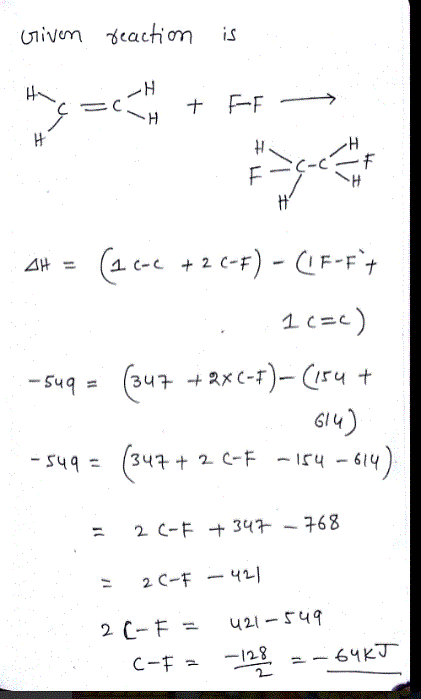# Question & Answer: Consider the following reaction: C2H4(g) + F2(g) → C2H4F2(g) ΔH = -549 kJ Estimate the…..

Consider the following reaction: C2H4(g) + F2(g) → C2H4F2(g) ΔH = -549 kJ Estimate the carbon-fluorine bond energy given that the C−C bond energy is 347 kJ/mol, the C=C bond energy is 614 kJ/mol, and the F−F bond energy is 154 kJ/mol.